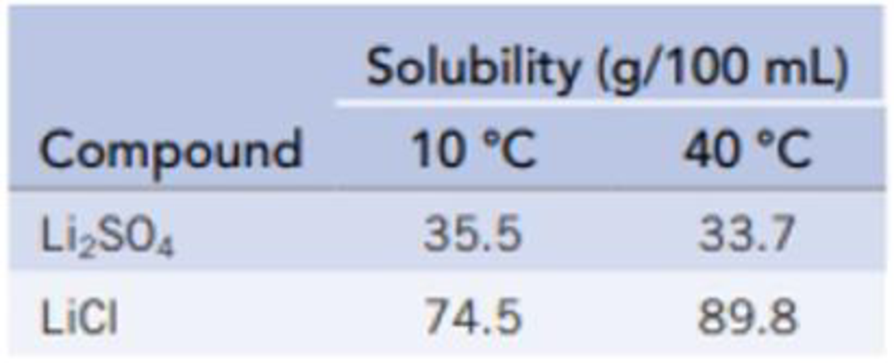Chapter 13, Problem 18PS

Chapter
Section
Textbook Problem

Some lithium chloride, LiCl, is dissolved in 100 mL of water in one beaker, and some Li2SO4 is dissolved in 100 mL of water in another beaker. Both are at 10 °C, and both are saturated solutions; some solid remains undissolved in each beaker. Describe what you would observe as the temperature is raised. The following data are available to you from a handbook of chemistry:Interpretation Introduction

Interpretation: The observation when the temperature is increased in the given condition has to be identified.

Concept introduction:

Solubility: The concentration of solute in a saturated solution in equilibrium with undissolved solute.

Explanation

The solubility of Li2SO4 decreases as the temperature is raised whereas the solubility of LiCl increases as the temperature is raised. The reason lies in the size of the ionic particles. When the size of the solute is larger, then it is difficult for the solvent molecules to surround bigger molecules

Still sussing out bartleby?

Check out a sample textbook solution.

See a sample solution

The Solution to Your Study Problems

Bartleby provides explanations to thousands of textbook problems written by our experts, many with advanced degrees!

Get Started